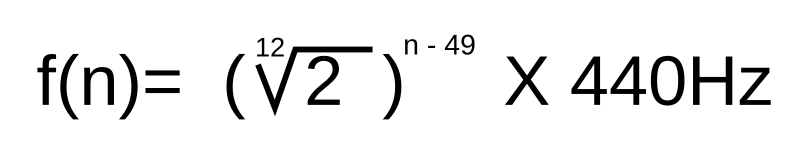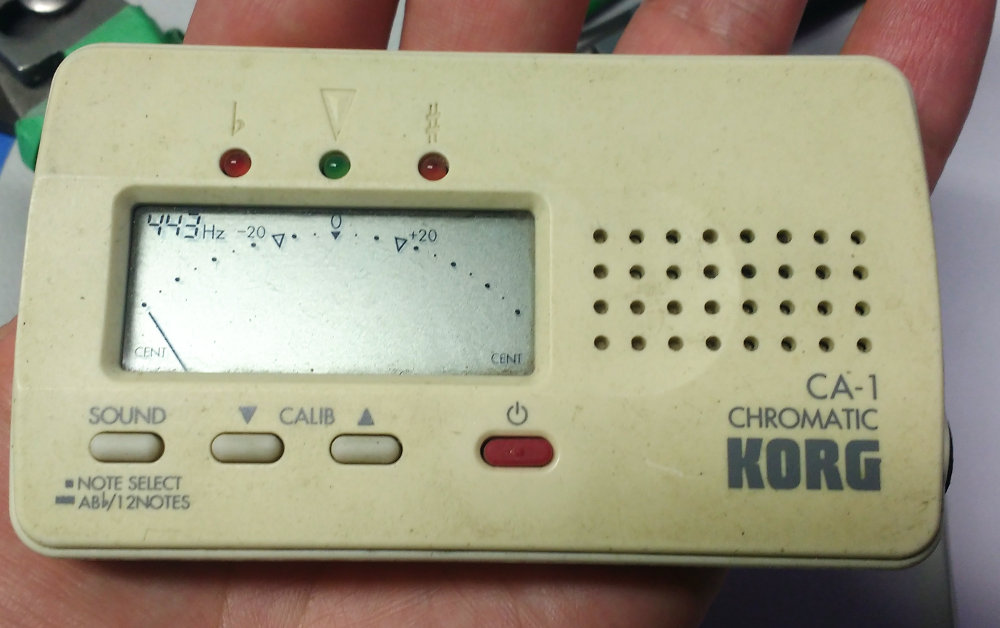# Blogs

## How many cents are in a Hz?

"How many cents are there in a Hz?"

That's a great question.

Some folks say about four. They are correct. They are giving you the information that you need to know about tuning a harp.

Every Hz you increase the reference pitch on your tuner (A=440 Hz) means you are raising each and every note it reads by about four cents.

(You can stop reading now unless you are feeling cheeky in a scientific way.)

But some folks will tell you that the frequency (in Hz) of notes changes on a logarithmic scale - the frequency doubles for every octave we go up.

Here is the formula for frequency of a note based on it's placement on the piano keyboard. A4 (or "A above Middle-C") is the 49th note. We use it as a reference.These folks tell you that one octave below middle C (C3), one cent equals 0.075 Hz and one octave above middle C (C5), one cent = 0.302 Hz. THEY ARE NOT THE SAME!

Sure, they are correct, but this is NOT useful information you need to know to tune a harp.

WHY?

Because we are not asking how many Hz there are in a cent. We are asking how many cents there are in a Hz.

Not just any Hz. We are asking by how many cents are we offsetting the note when we change our reference from A=440 Hz to A=441 Hz."Tune to 443" means tune your instrument using 443 Hz as the pitch for A above Middle C.

It does NOT mean tune every note up by three Hz. Why?

- We tune using cents, not Hz
- One cent is different in every octave
- Our tuner does the conversion for us

THE BOTTOM LINE: When you adjust the base frequency (440 Hz) on a chromatic tuner by one Hz, you are adjusting all the other notes of the scale by the same relative value. That happens to be four cents, no matter how many Hz there are in any of the notes' cents.

How many Hz in a cent doesn't really matter - we are only talking about making a change in the reference. Your tuner does the heavy lifting and instantly identifies the note (along with the offset in cents) of the frequency it is hearing.

## Pages